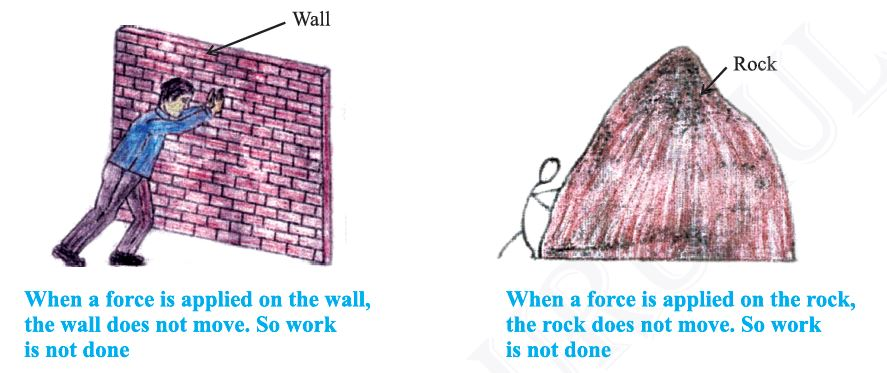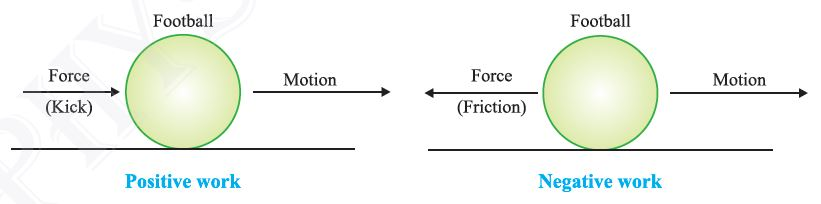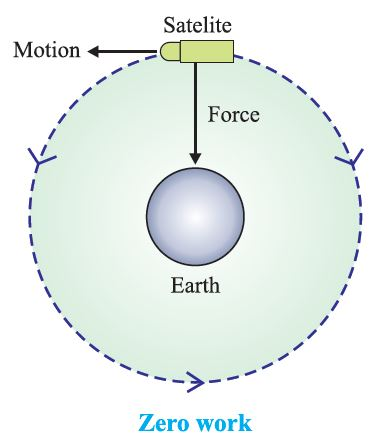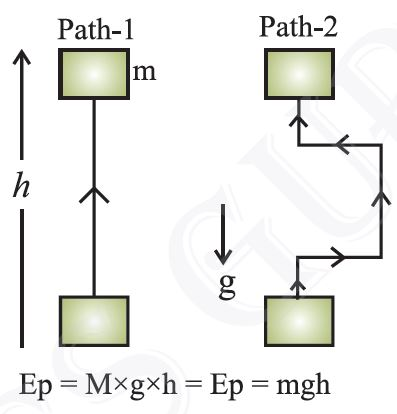# Work & EnergyWork

For doing work, energy is required.
• In animals, energy is supplied by food they eat.
• In machine, energy is supplied by fuel.

Not much work inspite of working hard : Reading, writing, drawing, thinking, analysing are all energy consuming. But in scientific manner, no work is done in above cases.

• Example :

• A man is completely exhausted in trying to push a rock (wall), but work done is zero as wall is stationary.

• A man standing still with heavy suitcase may be tired soon but he does no work in this situation as he is stationary.Work is said to be done when :
(i) a moving object comes to rest.
(ii) an object at rest starts moving.
(iii) velocity of an object changes.
(iv) shape of an object changes.

Scientific Conception of Work
• Work is done when a force produces motion in a body.
• Work is said to be done when a force is applied on a body and the body moves under the influence of force.

Condition of Work
(i) Force should be applied on the body.
(ii) Body should be displaced.Examples :

Work is done when :
(i) A cyclist is pedaling the cycle.
(ii) A man is lifting load in upward or downward direction.

Work is not done when :
(ii) A man is applying force on a big rock.

Work Done by a Fixed Force
Work done in moving a body is equal to the product of force and displacement of body in the direction of force.Work = Force × Displacement
W = F × S
Work is a scalar quantity.

Unit of Work

Unit of work is Newton metre or Joule.

When a force of 1 Newton moves a body through a distance of 1 metre in its own direction, then the work done is 1 Joule.
1 Joule = 1 Newton × 1 metre
1 J = 1 NmThe amount of work done depends on the following factors :

(i) Magnitude of force : Greater the force, greater is the amount of work & vice versa.

(ii) Displacement : Greater the displacement, greater is the amount of work & vice-versa.

Negative, Positive and Zero Work

Work done by a force can be positive, negative or zero.
(i) Work done is positive when a force acts in the direction of motion of
the body.[ Fig. (a)] (θ = 0º).

Example :

A child pulls a toy car with a string horizontally on the ground.

Here work done is positive.
W = F × S(ii) Work done is negative when a force acts opposite to the direction of motion of the body.

Example : When we kick a football lying on the ground, the force of our kick
moves the football. Here direction of force applied & motion of football is same
so work done is positive. But when football slows due to force of friction acting
in a direction opposite to direction of motion of football [Fig. (b)], work done is
negative.(iii) Work done is zero when a force acts at right angles to the direction of motion.

Example :

The moon moves around the earth in circular path. Here force of gravitation acts on the moon at right angles to the direction of motion of the moon. So work done is zero.Note that if work is done against the direction of motion (gravity), then it is taken –ve.

Example. A coolie lifts a luggage of 15 kg from the ground and put it on his head 1.5 m above the ground. Calculate the work done by him on the luggage.

Solution : Mass of luggage (m) = 15 kg
Displacement (S) = 1.5 m
So, Work done, W = F × S         [F = mg]
= 15 × 10 × 1.5 [g = 10 m/s^2]  [g = force of gravity]
= 225.0 kg m/s^2
= 225 N-m = 225 J
Hence, work done = 225 J.

Energy

(i) The sun is the biggest source of energy.
(ii) Most of the energy sources are derived from the sun.
(iii) Some energy is received from nucleus of atoms, interior of the earth and the tides.

Definition : The capacity of doing work is known as energy.

The amount of energy possessed by a body is equal to the amount of work it can do. Working body losses energy, body on which work is done gains energy.

Energy is a scalar quantity.

Unit : The SI unit of energy is Joule (J) and its bigger unit is kilo joule (kJ).
1 kJ = 1000 J
The energy required to do 1 Joule of work is called 1 Joule energy.

Forms of Energy

Main forms of energy are :

(i) Kinetic energy

(ii) Potential energy

(iii) Heat energy

(iv) Chemical energy

(v) Electrical energy

(vi) Light energy

(vii) Sound energy

(viii) Nuclear energy

• Sum of kinetic energy & potential energy of a body is called mechanical energy.

Mechanical energy

The energy possessed by a body on account of its motion or position is called mechanical energy.

Kinetic Energy

The energy of a body due to its motion is called kinetic energy.

Examples of kinetic energy :

• A moving cricket ball
• Running water
• A moving bullet
• Flowing wind
• A moving car
• A running athelete
• A rolling stone• Flying aircraft

Formula for Kinetic Energy

If an object of mass ‘m’ moving with uniform velocity ‘u’, it is displaced through a distance ‘s’. Constant force ‘f’ acts on it in the direction of displacement. Its velocity changes from ‘u’ to ‘v’. Then acceleration is ‘a’.

Work done, W= f x s  &  f=ma

according to IIIrd kinematical equation, relationship between u,v,s and a is as follows:

V2-u2=2as

s = (v2-u2)/2a

Now putting the value of f and s , we have

W = (ma) x (v2-u2)/2a = m (v2-u2)/2

If u = 0 (when body starts moving from rest)

W =  ½mv2

or, Kinetic Energy = ½mv2

Example. An object of mass 15 kg is moving with uniform velocity of 4 m/sec. What is the kinetic energy possessed by it ?

Solution : Mass of the object, m = 15 kg
Velocity of the object, v = 4 m/s
K.E. = ½mv2
= ½ × 15 kg × 4 m/s × 4 m/s
= 120 J
The kinetic energy of the object is 120 J.

Potential Energy

The energy of a body due to its position or change in shape is known as potential energy.

Examples :

(i) Water kept in dam : It can rotate turbine to generate electricity due to its position above the ground.

(ii) Wound up spring of a toy car : It possess potential energy which is released during unwinding of spring. So toy car moves.

(iii) Bent string of bow : Potential energy due to change of its shape (deformation) released in the form of kinetic energy while shooting an arrow.Factors affecting Potential Energy

(i) Mass : P. E. ∝ m
More the mass of body, greater is the potential energy and vice-versa.

(ii) Height above the ground :
P. E. ∝ h (Not depend on the path it follows)
Greater the height above the ground, greater is the P.E. and vice-versa.

(iii) Change in shape : Greater the stretching, twisting or bending, more is the potential energy.

Potential Energy of an Object on a Height

If a body of mass ‘m’ is raised to a height ‘h’ above the surface of the earth, the gravitational pull of the earth (m × g) acts in downward direction. To lift the body, we have to do work against the force of gravity.

Thus, Work done, W = Force × Displacement
Or W = m × g × h = mgh
This work is stored in the body as potential energy (gravitational potential
energy).

Thus, Potential energy, P.E. = m × g × h (where, g = acceleration due to gravity.)Example. If a body of mass 10 kg is raised to a height of 6 m above the earth, calculate its potential energy.

Solution :

Potential energy of the body = mgh
Mass of body = 10 kg
Height above the earth = 6 m
Acceleration due to gravity = 10 m/s^2
So, P.E. = 10 × 10 × 6  = 600 J
Thus, potential energy of the body is 600 Joules.

Transformation of Energy

The change of one form of energy to another form of energy is known as transformation of energy.

Example :

(i) A stone on a certain height has entire potential energy. But when it starts moving downward, potential energy of stone goes on decreasing as height goes on decreasing but its kinetic energy goes on increasing as velocity of stone goes on increasing. At the time stone reaches the ground, potential energy becomes zero and kinetic energy is maximum.
Thus, its entire potential energy is transformed into kinetic energy.

(ii) At hydroelectric power house, the potential energy of water is transformed into kinetic energy and then into electrical energy.

(iii) At thermal power house, chemical energy of coal is changed into heat energy, which is futher converted into kinetic energy and electrical energy.

(iv) Plants use solar energy to make chemical energy in food by the process of photosynthesis.

Law of Conservation of Energy

• Whenever energy changes from one form to another form, the total amount of energy remains constant.

• “Energy can neither be created nor be destroyed.”

• Although some energy may be wasted during conversion, but the total energy of the system remains the same.

Conservation of Energy during Free Fall of a Body

• A ball of mass ‘m’ at a height ‘h’ has potential energy = mgh.

• As ball falls downwards, height ‘h’ decreases, so the potential energy also decreases.

• Kinetic energy at ‘h’ is zero but it is increasing during falling of ball.

• The sum of potential energy & kinetic energy of the ball remains the same at every point during its fall.
½mv2 + mgh = Constant
Kinetic energy + Potential energy = Constant

Rate of Doing Work – Power

“Power is defined as the rate of energy consumption.”

Power = work done / time

or, P= W/t
where,  P = Power
W = Work done
t = Time taken

Unit of Power
SI unit of Power is Watt (W) = 1 Joule/second.
1 Watt = 1 J / 1 s

Power is 1 Watt when 1 joule work is done in one second.

Average Power = Total work done or total energy used / Total time taken

The power of an electrical appliance tells us the rate at which electrical energy
is consumed by it.

Bigger unit of Power : Bigger unit of power is called Kilowatt or KW.
1 Kilowatt (KW) = 1000 Watt = 1000 W or 1000 J/s

Example. A body does 20 Joules of work in 5 seconds. What is its power ?

Solution :

Power = Work done / Time taken
Work done = 20 Joules
Time taken = 5 sec.
So, Power = 20 J / 5 s = 4 J/s = 4 W
Thus, power of the body is 4 Watts.

Commercial Unit of Energy : Joule is very small unit of energy and it is inconvenient to use it where a large quantity of energy is involved.

For commercial purpose, bigger unit of energy is Kilotwatt hour (KWh).

1 KWh : 1 KWh is the amount of energy consumed when an electric appliance having a power rating of 1 Kilowatt is used for 1 hour.

Relation between Kilowatt hour and Joule

1 Kilowatt hour is the amount of energy consumed at the rate of 1 Kilowatt for 1 hour.

1 Kilowatt hour = 1 Kilowatt for 1 hour
= 1000 Watt for 1 hour
= 1000 Watt × 3600 seconds (60 × 60 seconds = 1 hour)
= 36,00,000 Joules

So, 1 KWh = 3.6 × 106 J = 1 unit

Example. A bulb of 60 Watt is used for 6 hrs. daily. How many units (KWh) of electrical energy are consumed ?

Solution : Power of bulb = 60 W = 60 KW /1000 = 0.06 KW

t = 6 hours
Energy = Power × Time taken = 0.06 × 6 h
= 0.36 KWh = 0.36 units